# NCERT Exemplar Solutions for Class 11 Maths Chapter 9 Sequence and Series

The solutions PDF is an important reference guide to help students score well in Class 11 examinations. Solving exercise-wise problems daily helps them improve their problem-solving skills, which are important to obtain a better score. NCERT Exemplar Solutions for Class 11 Maths Chapter 9 Sequence and Series contain solutions to the problems provided in the NCERT Exemplar textbook. The questions from every section are solved accurately by our subject experts. NCERT Exemplar Solutions for Class 11 are detailed and step-by-step, to clear all the queries of the students. The exercise present in the chapter should be dealt with utmost sincerity if one wants to score high in the subject.

The 9th Chapter, Sequence and Series of NCERT Exemplar Solutions for Class 11 Maths, explains arithmetic and geometric progression. The main aim of formulating these solutions by BYJU’S subject experts is to strengthen learners’ fundamentals of Maths and thereby understand every concept clearly. Some of the essential topics covered in this chapter are listed below.

• Arithmetic progression – nth term formula, and the sum of n terms formula
• Geometric progression – nth term formula, geometric mean and the sum of n terms formula
• Important results on the sum of special sequences

## Download the PDF of NCERT Exemplar Solutions for Class 11 Maths Chapter 9 Sequence and Series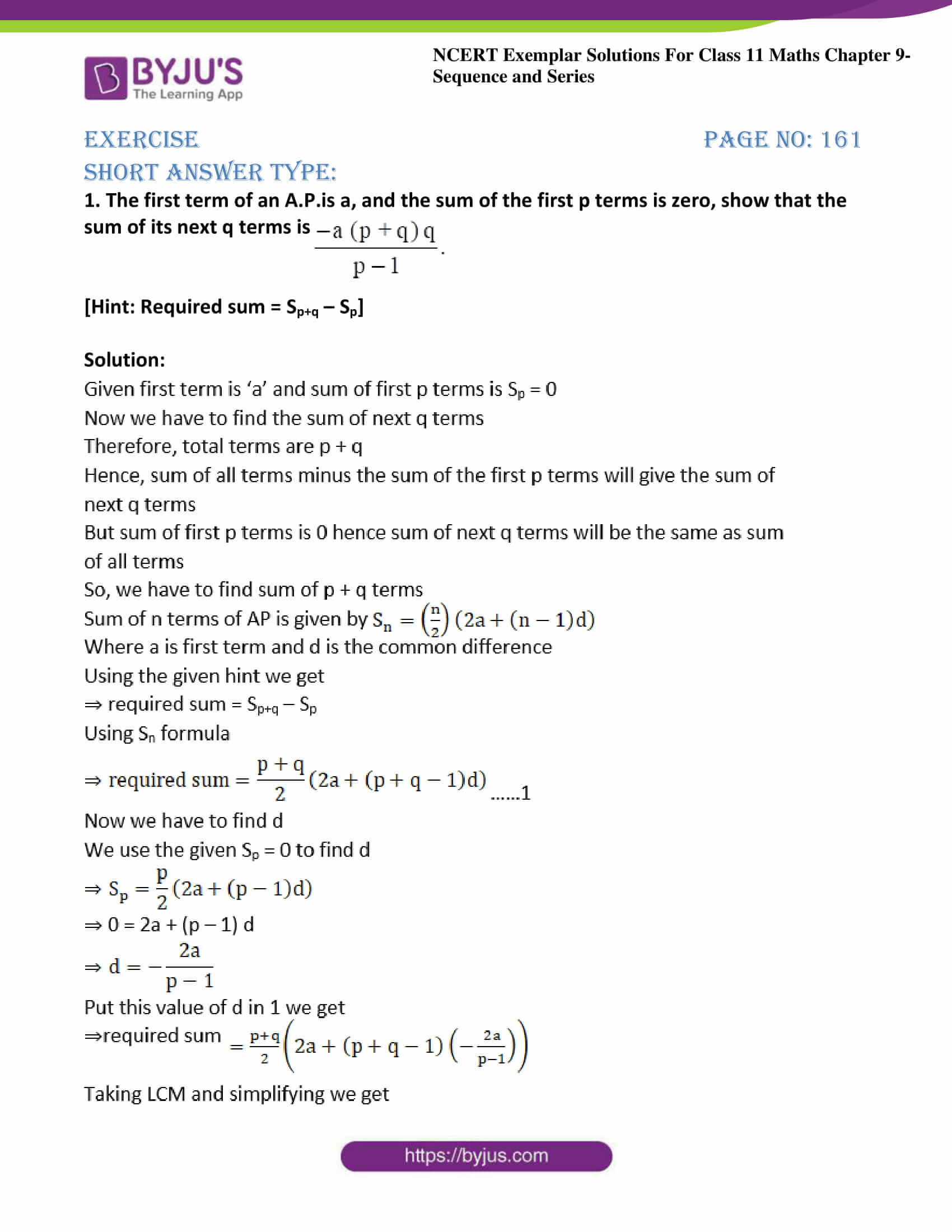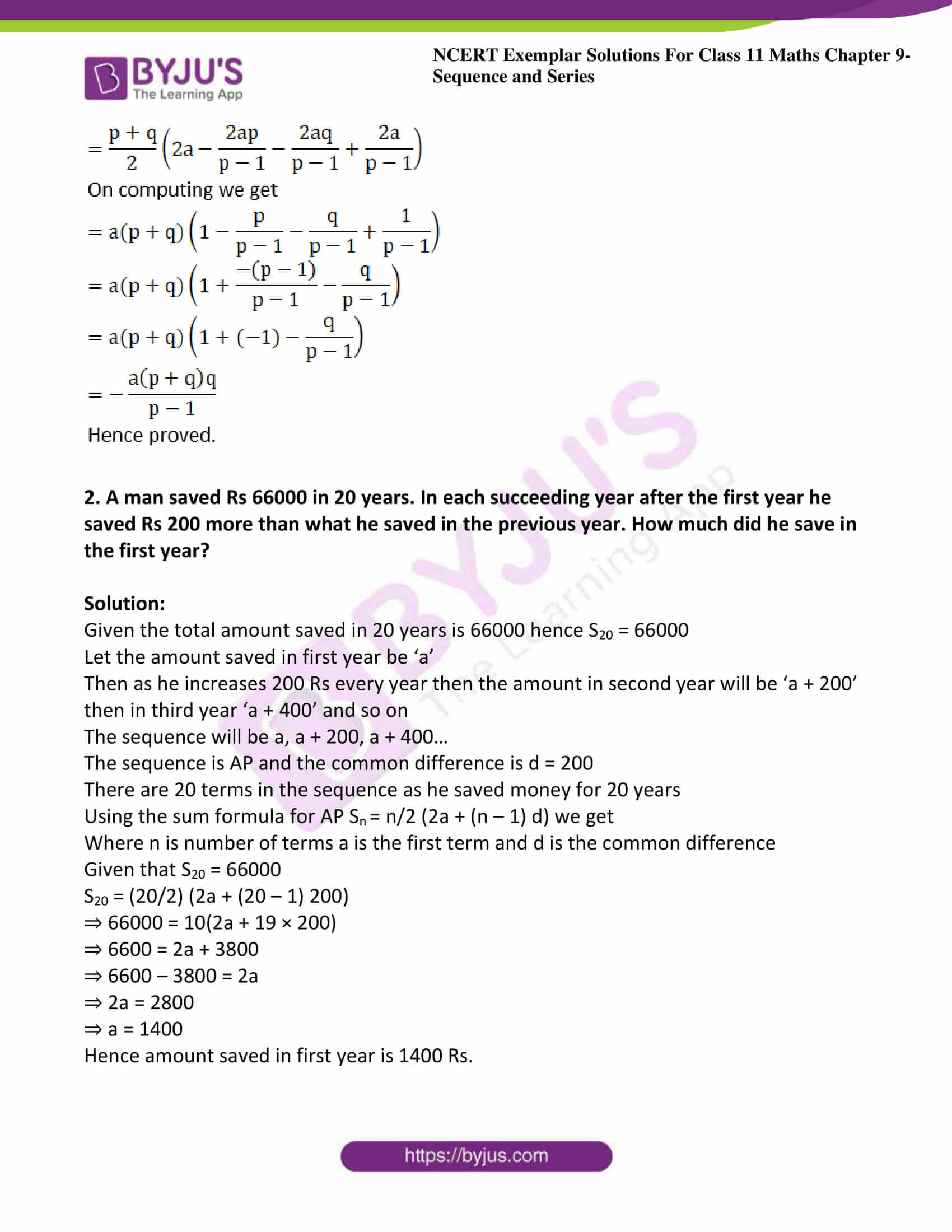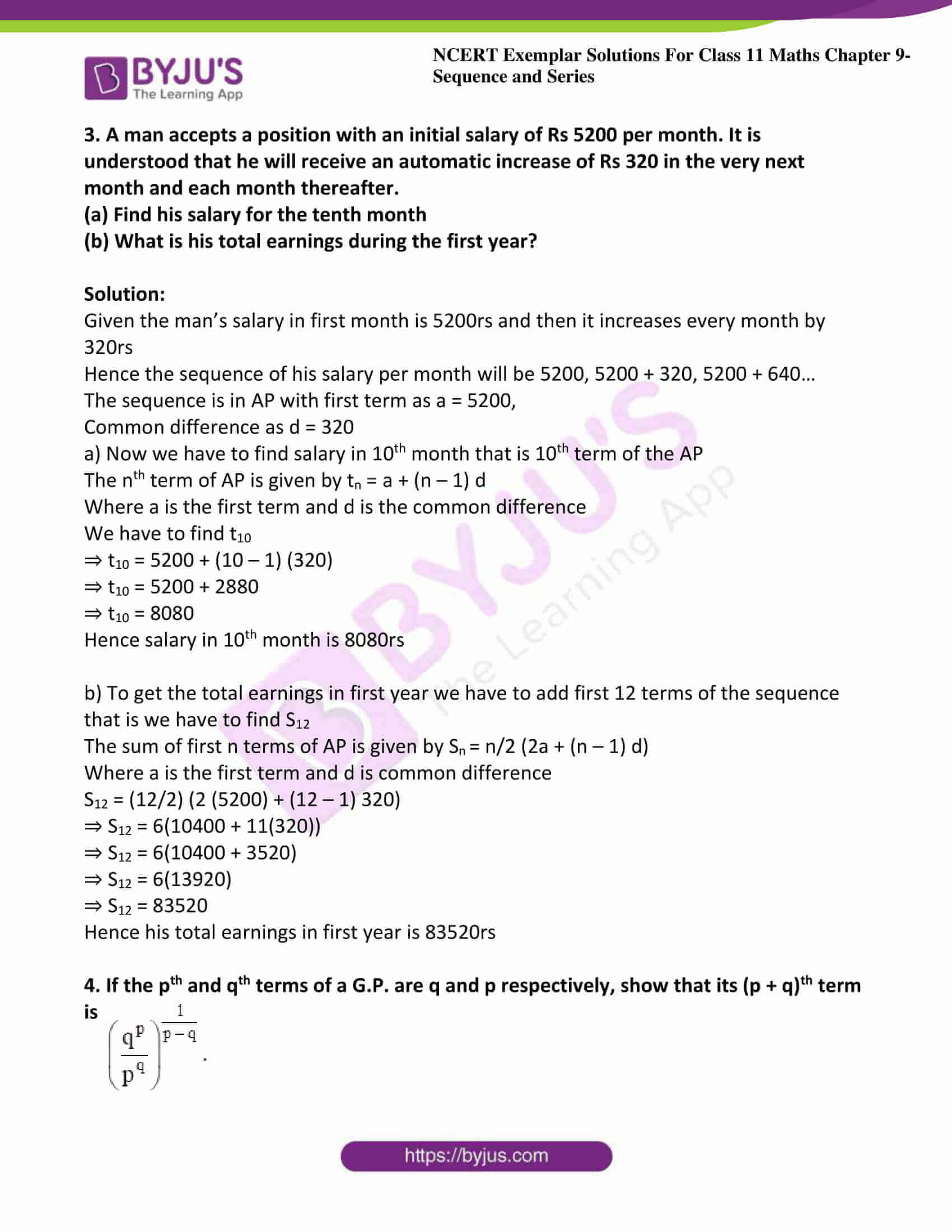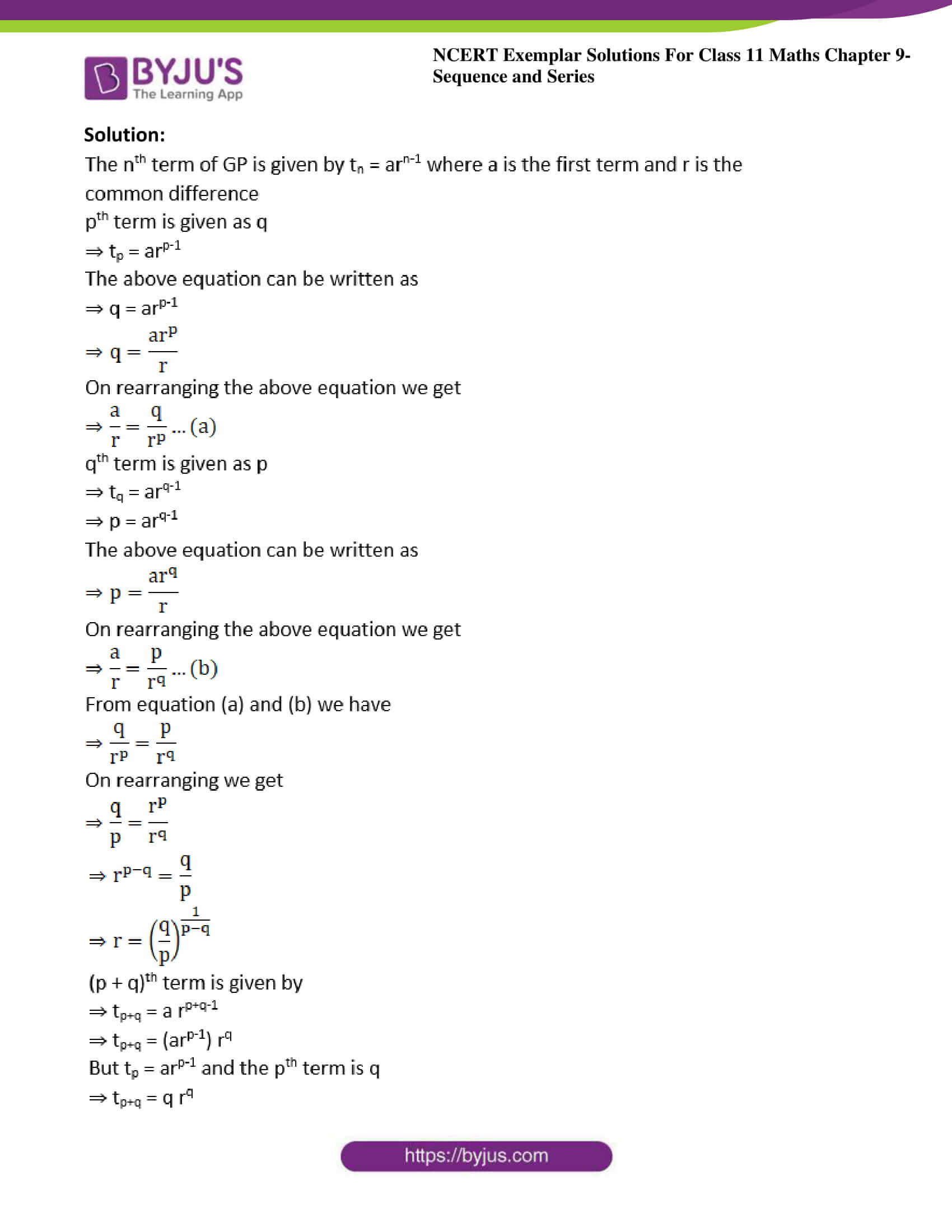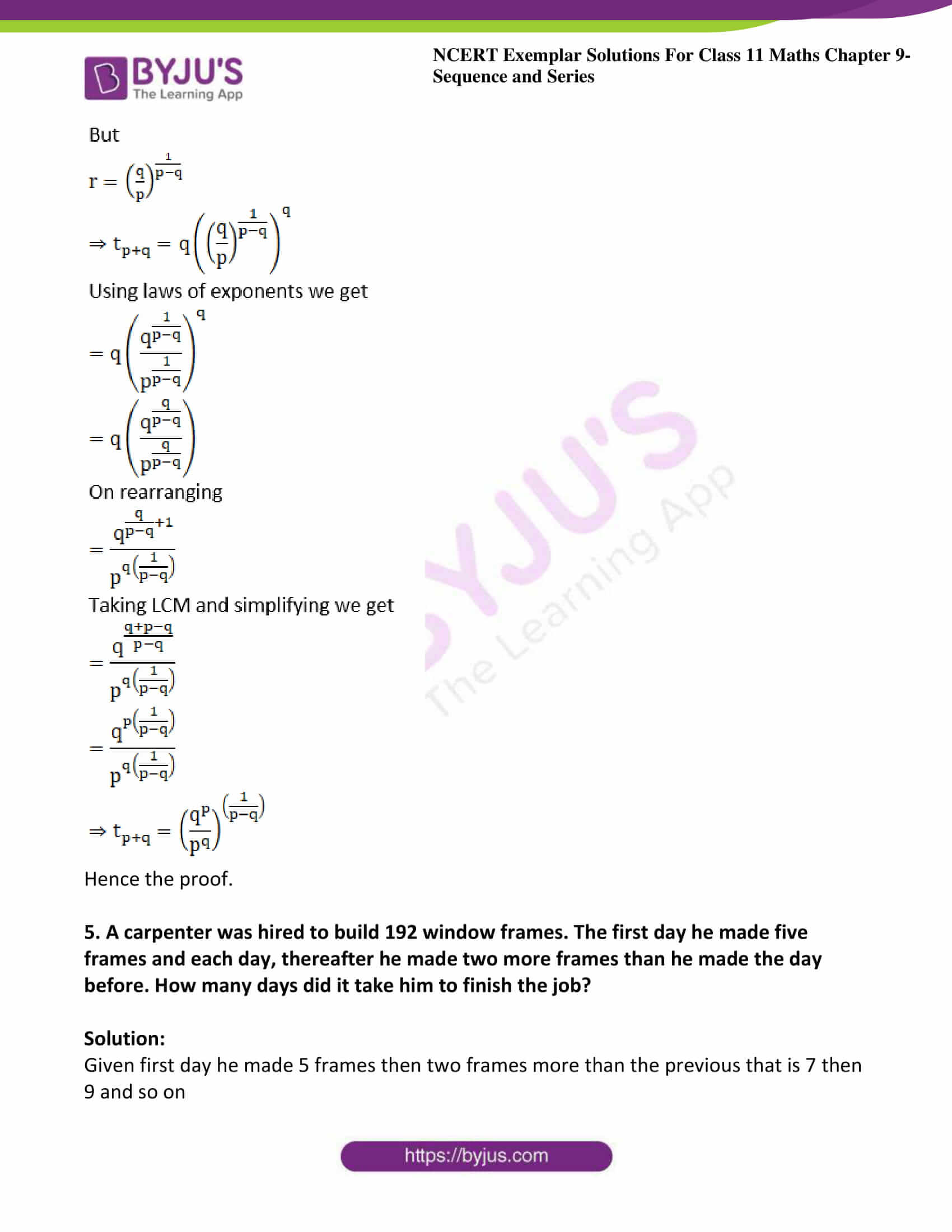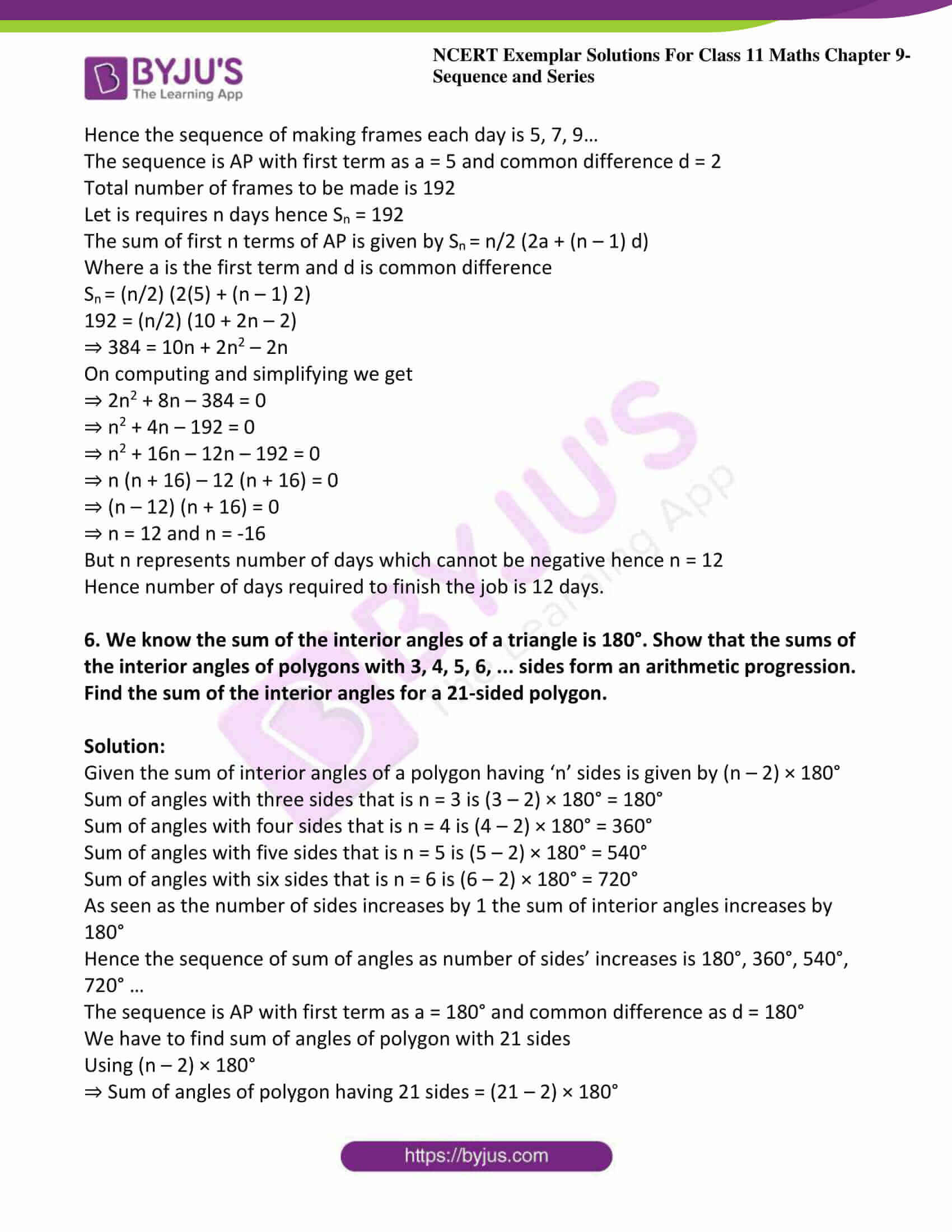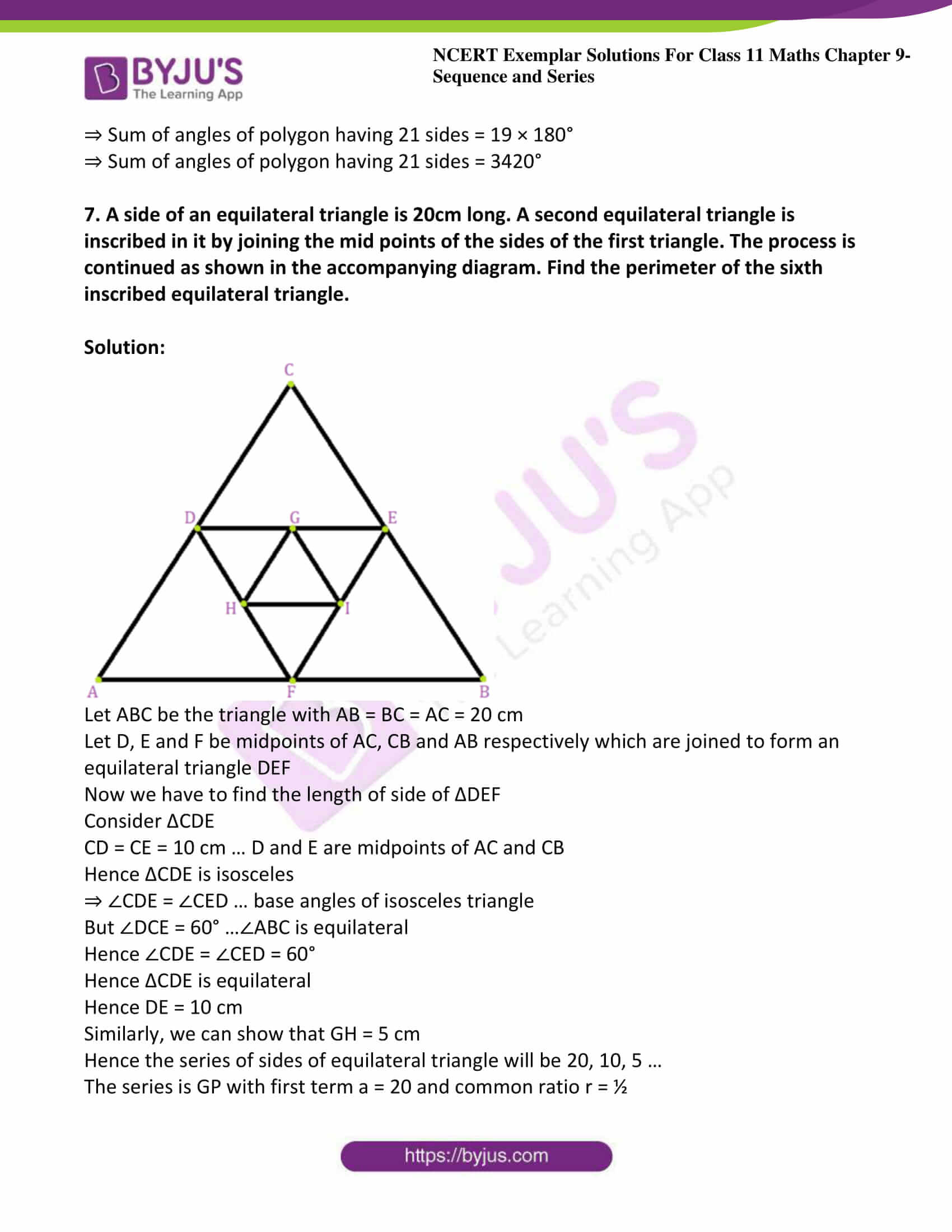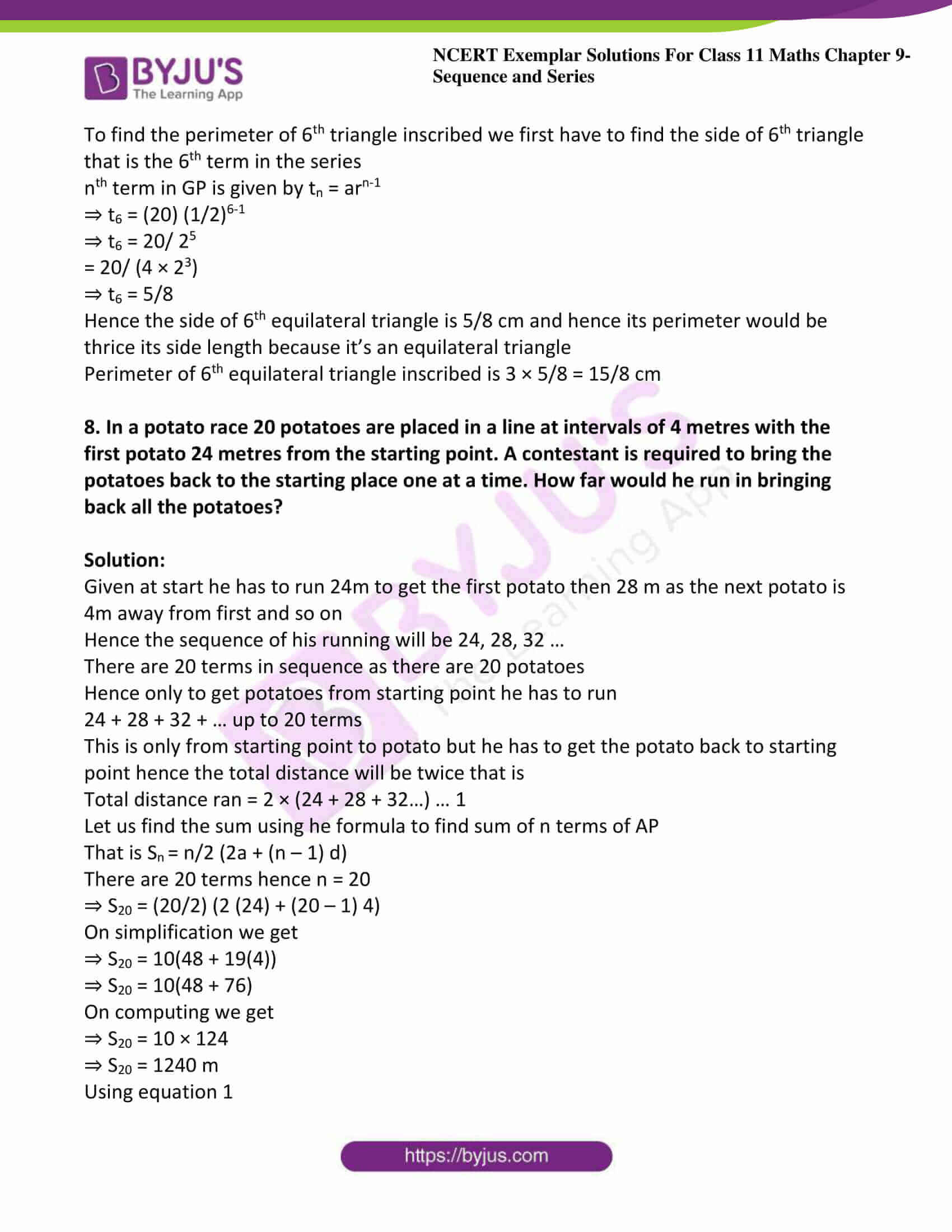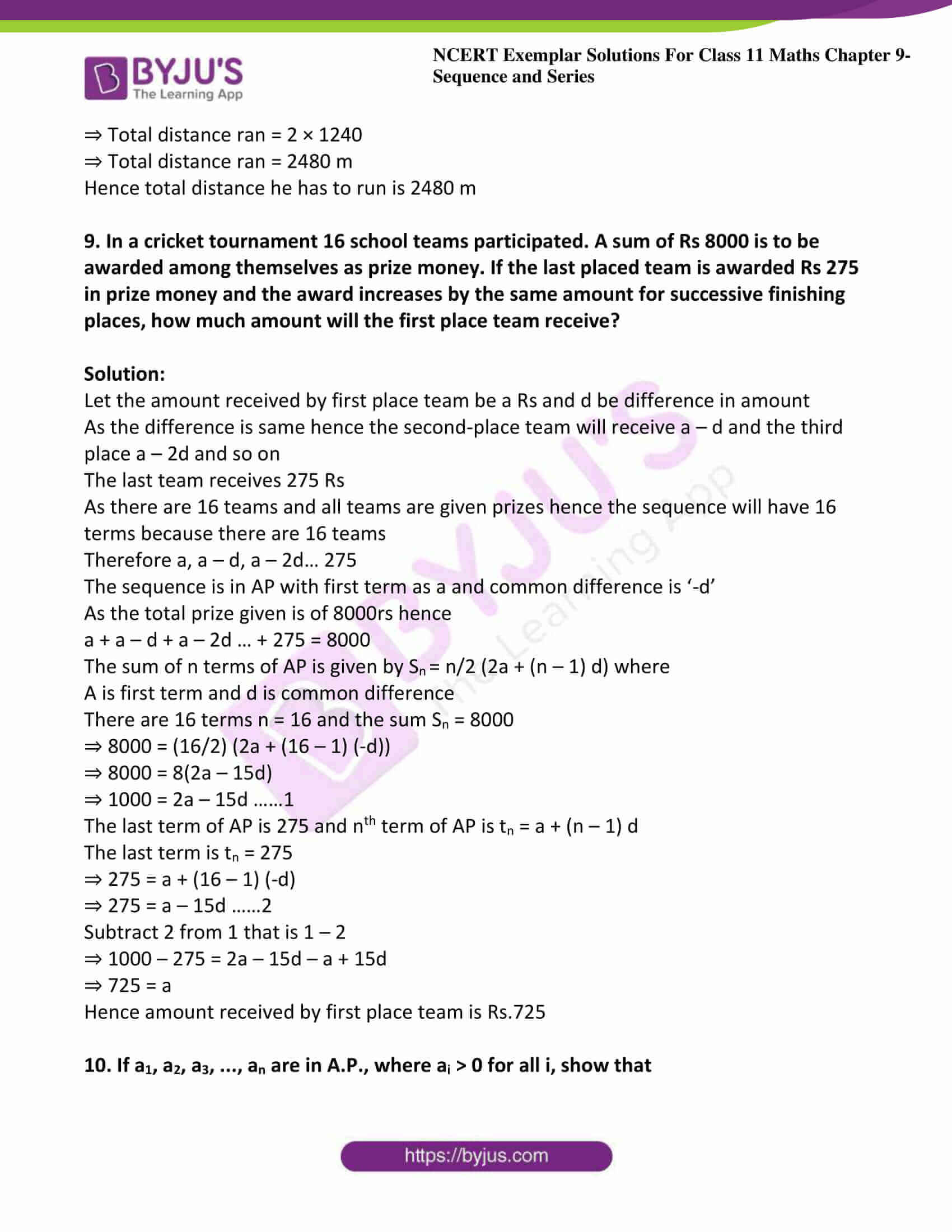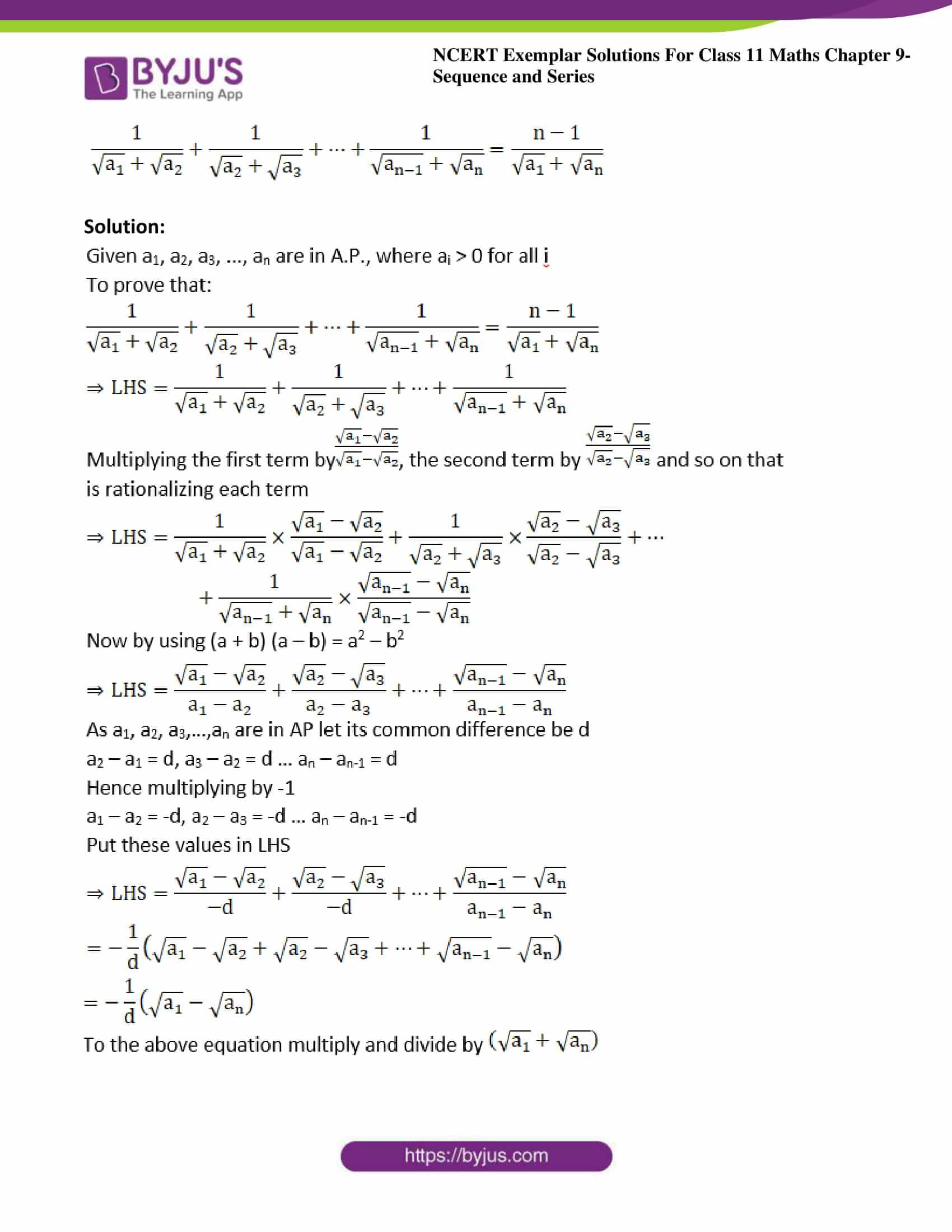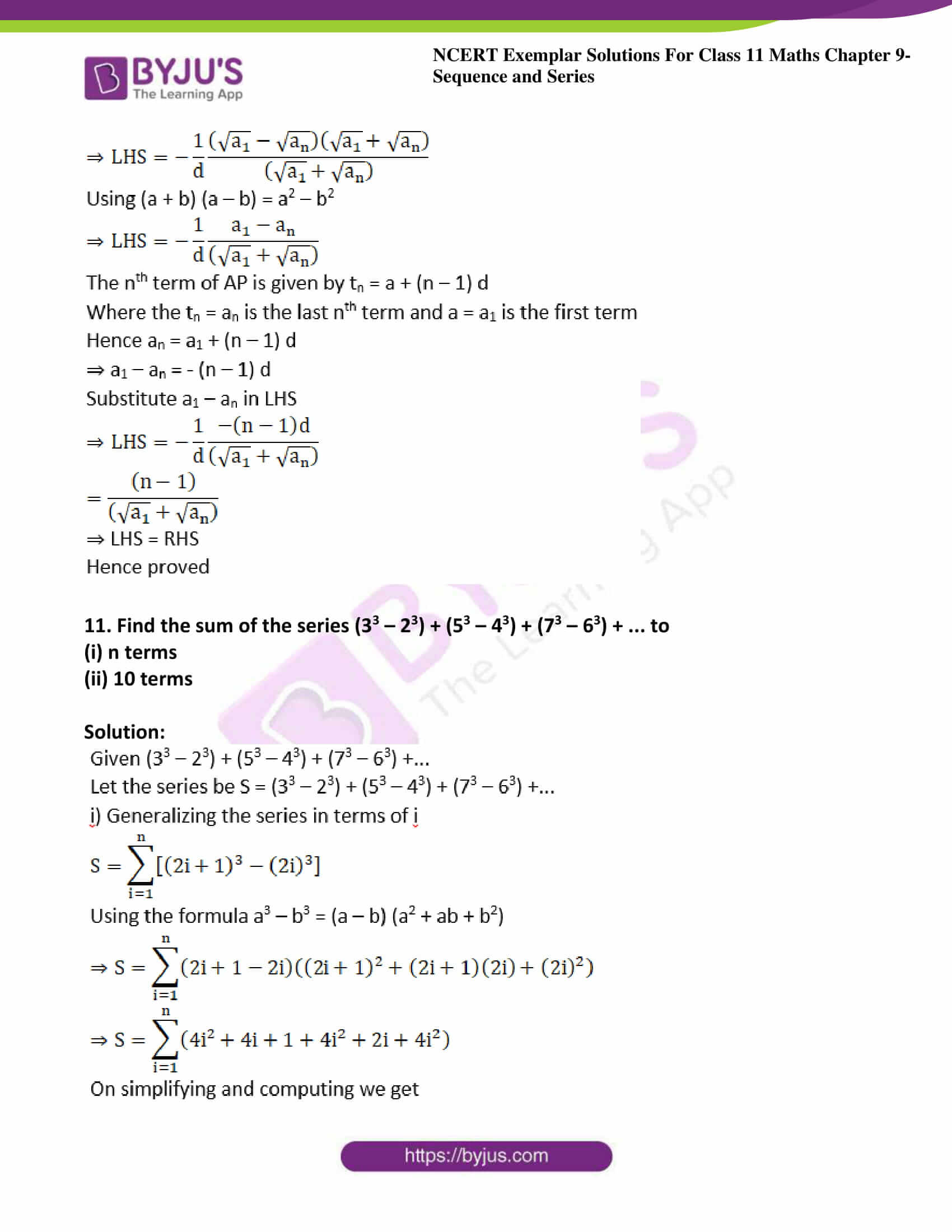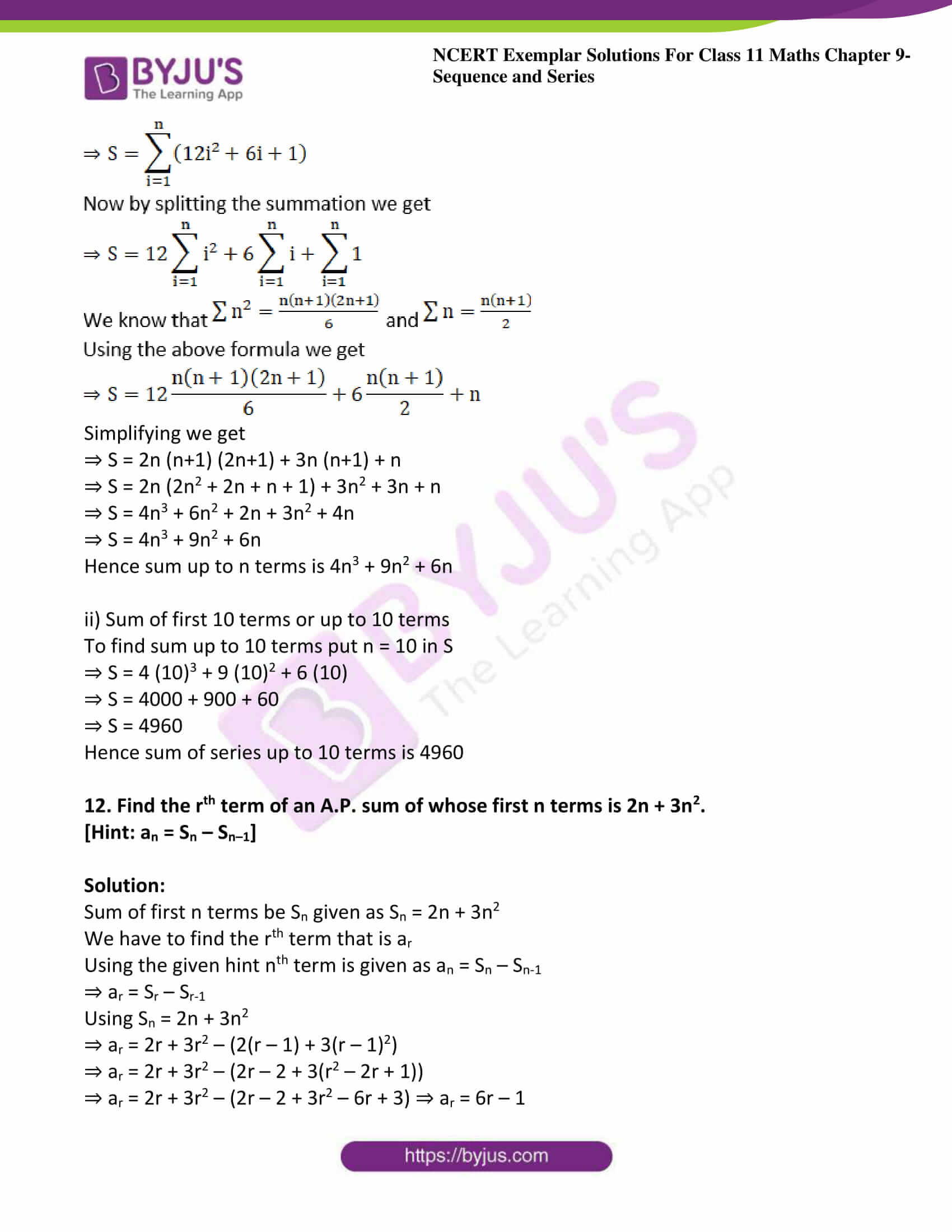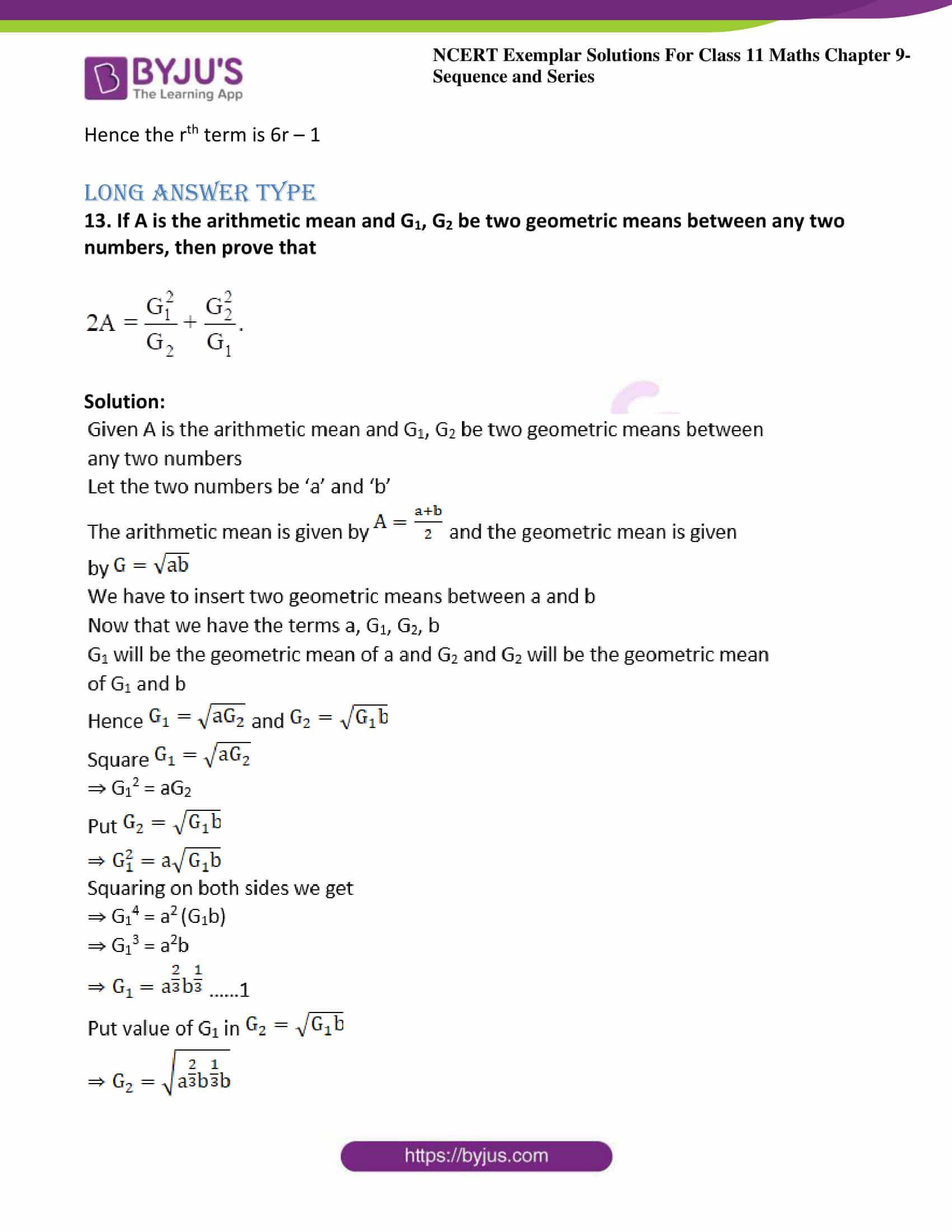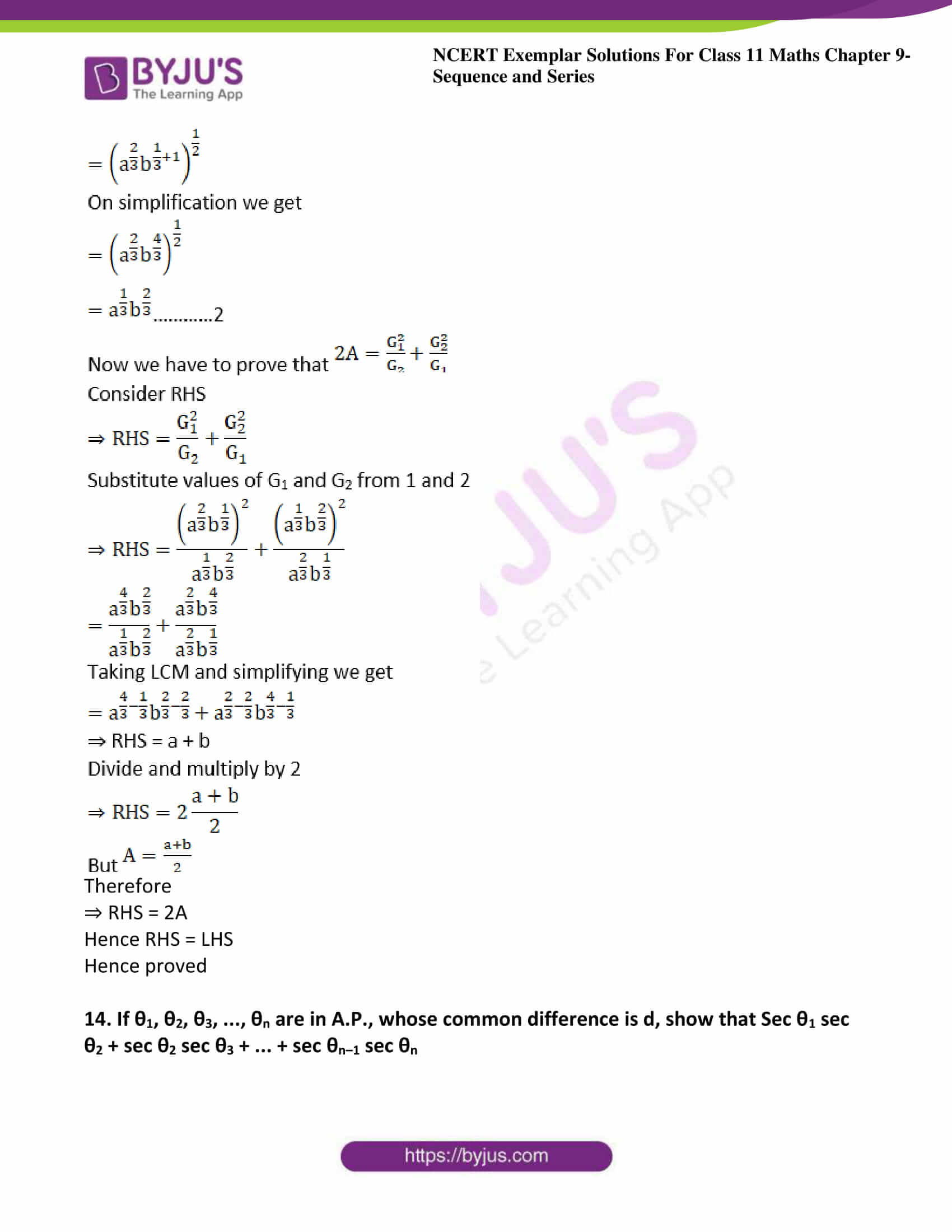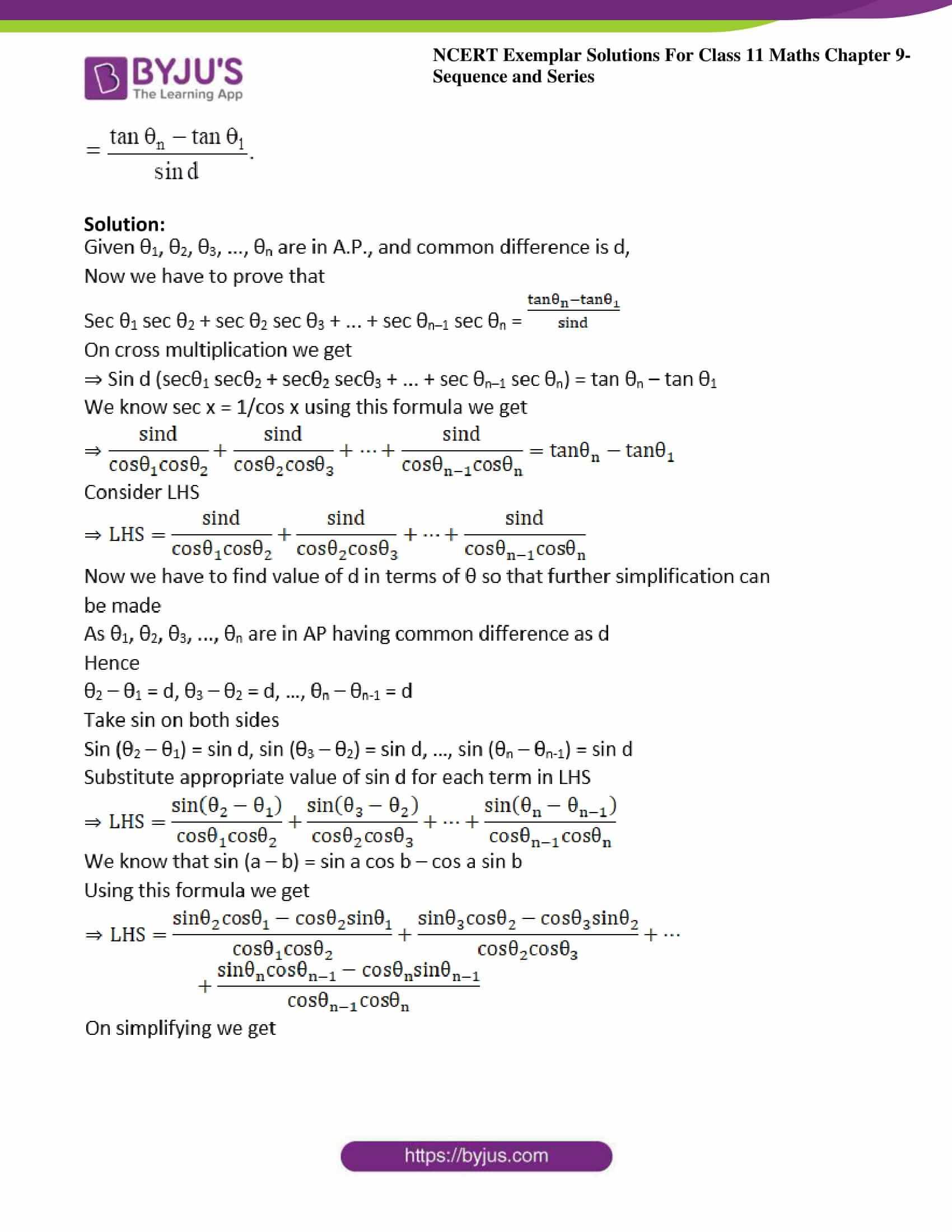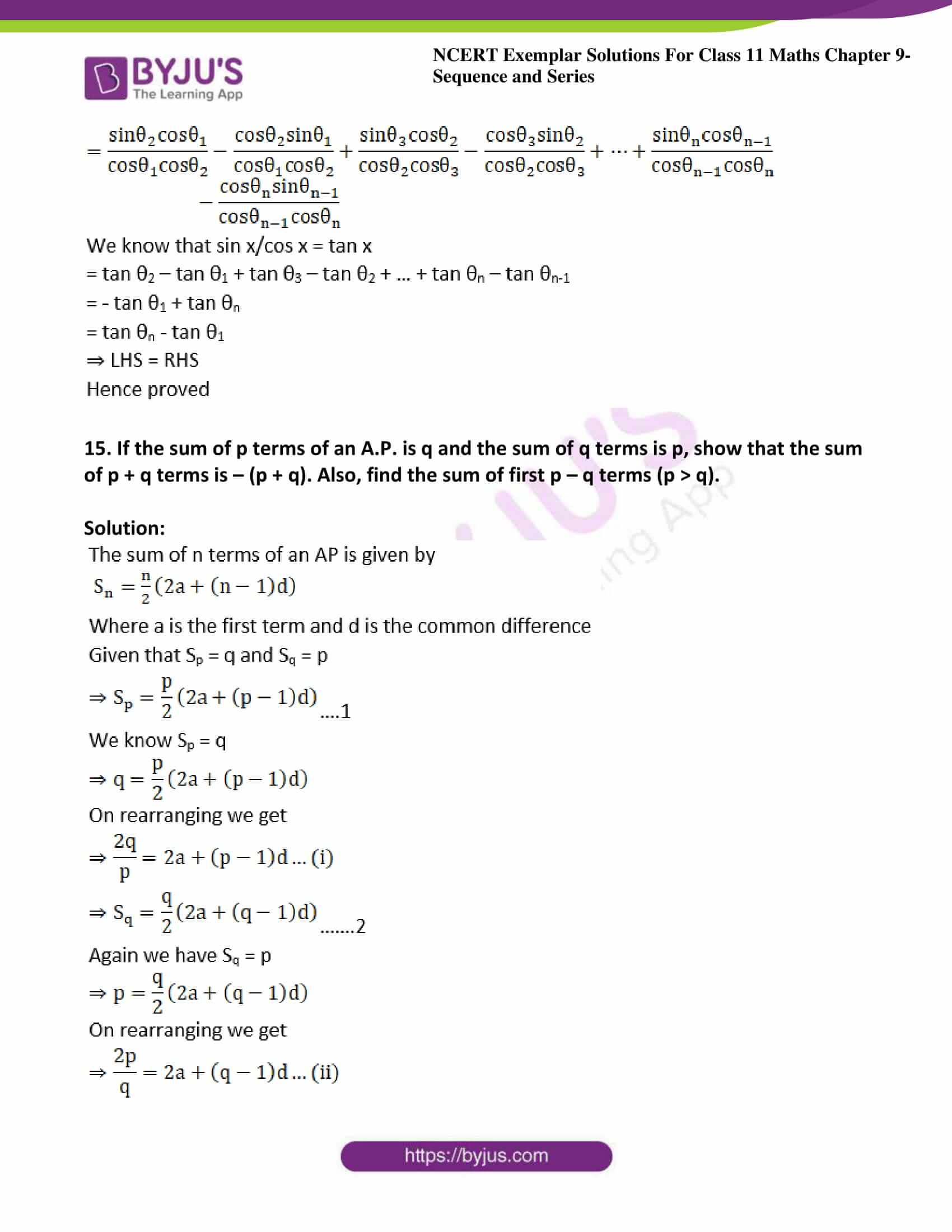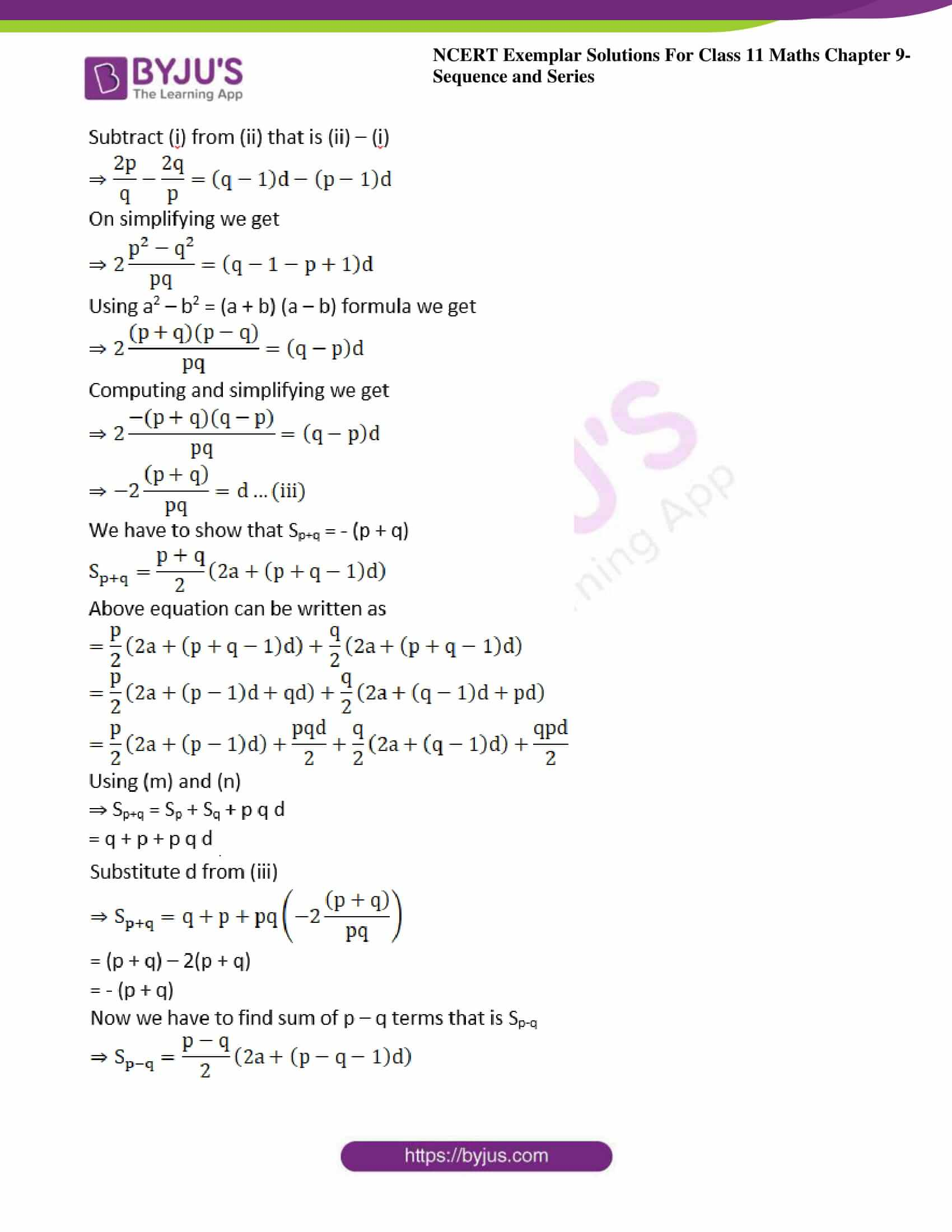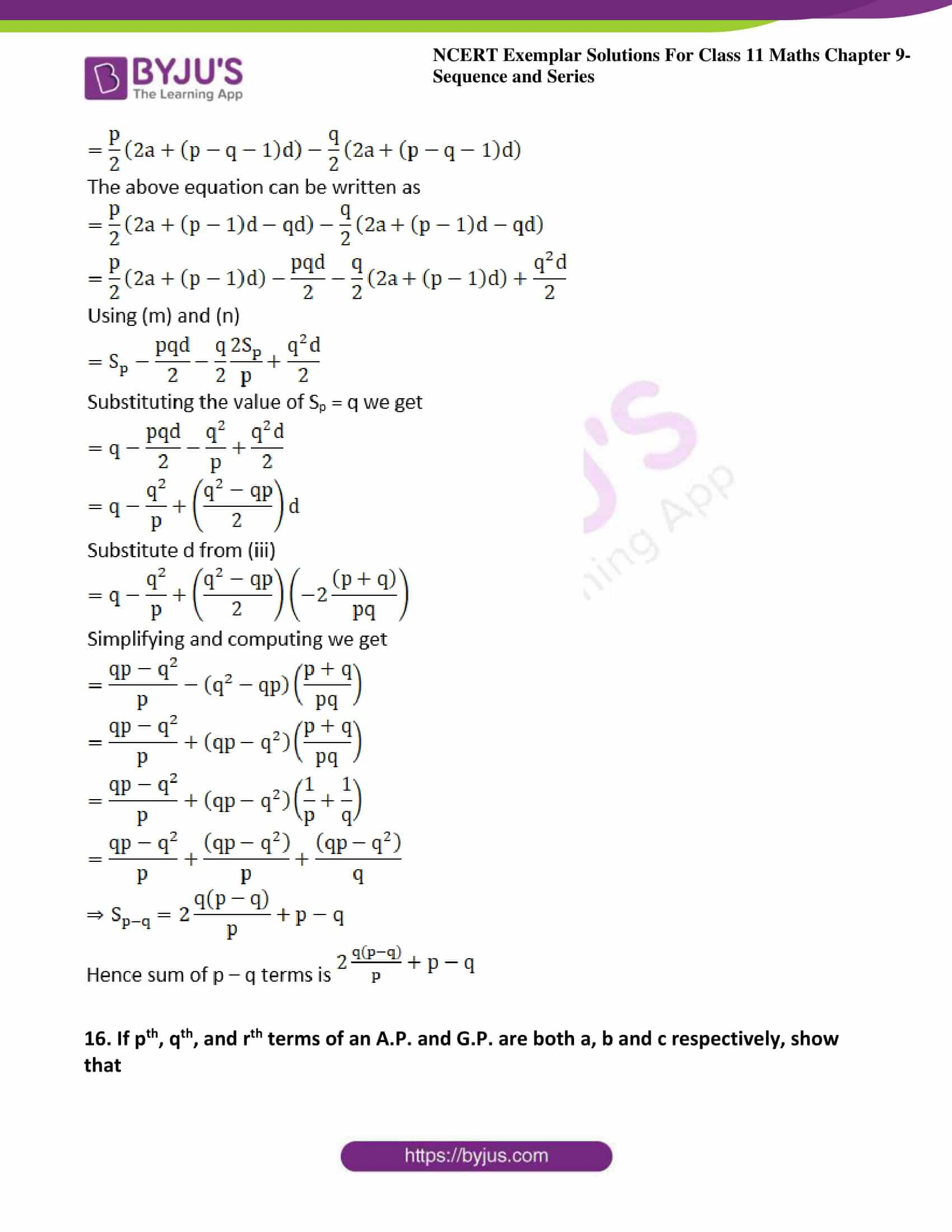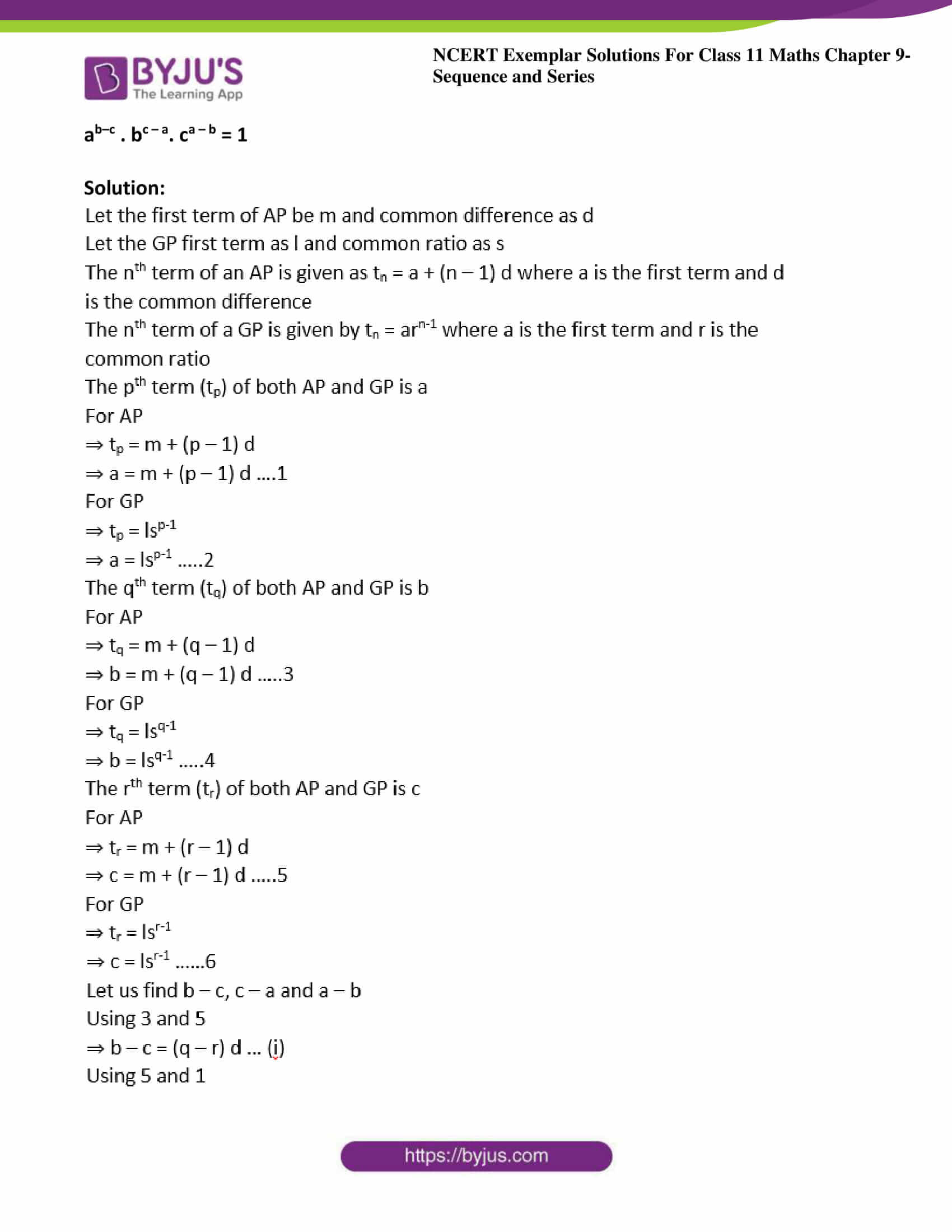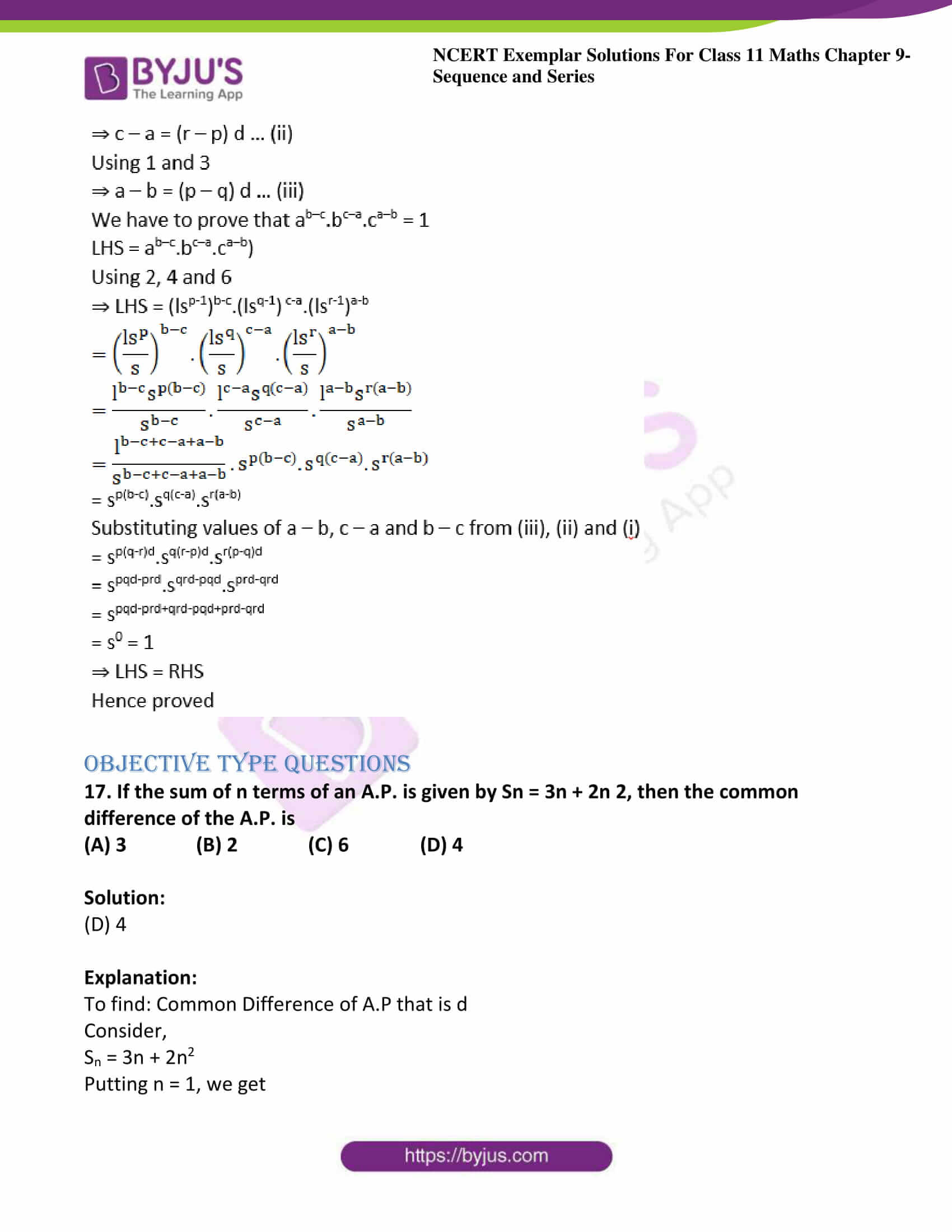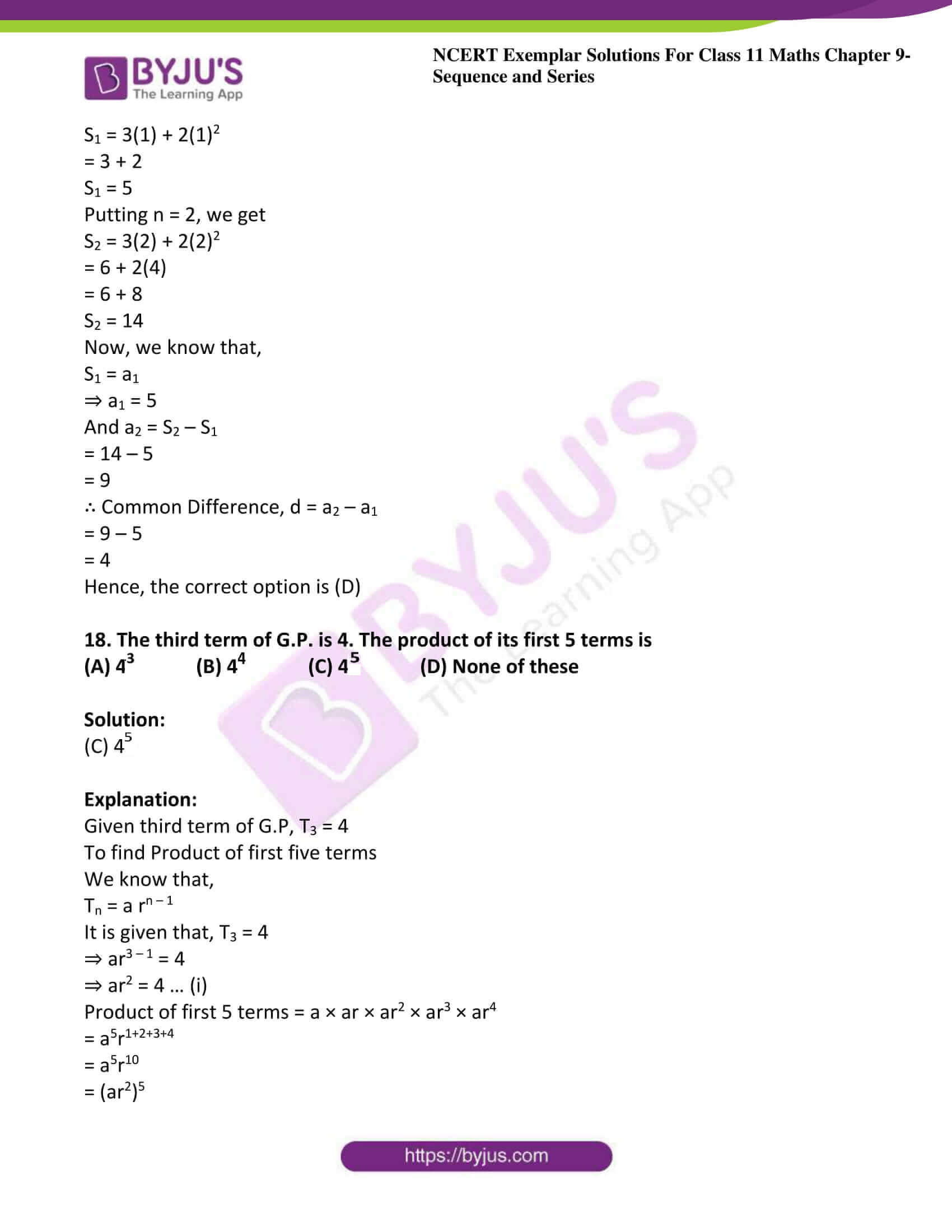### Access Answers to the NCERT Exemplar Class 11 Maths Chapter 9 – Sequence and Series

Exercise Page No: 161

1.The first term of an A.P.is a, and the sum of the first p terms is zero, show that the sum of its next q terms is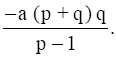[Hint: Required sum = Sp+q – Sp]

Solution: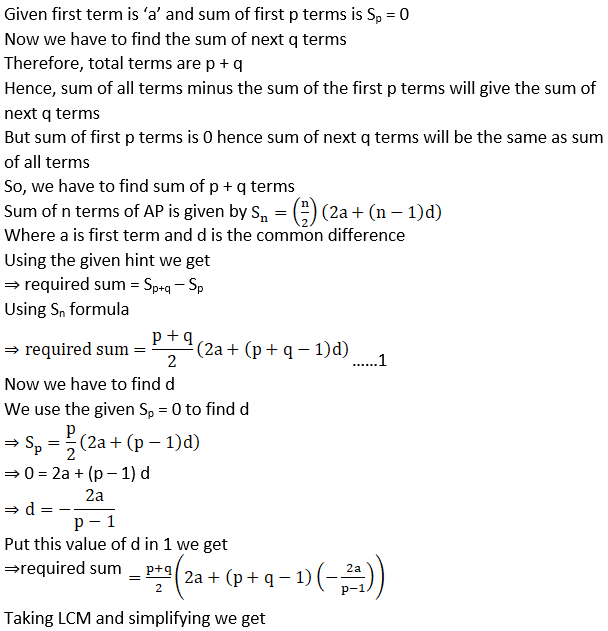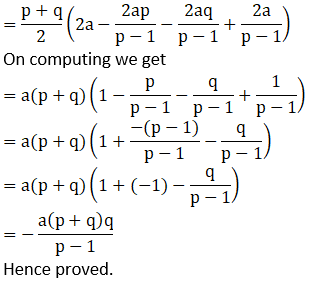2. A man saved Rs 66000 in 20 years. In each succeeding year after the first year he saved Rs 200 more than what he saved in the previous year. How much did he save in the first year?

Solution:

Given the total amount saved in 20 years is 66000 hence S20 = 66000

Let the amount saved in first year be ‘a’

Then as he increases 200 Rs every year then the amount in second year will be ‘a + 200’ then in third year ‘a + 400’ and so on

The sequence will be a, a + 200, a + 400…

The sequence is AP and the common difference is d = 200

There are 20 terms in the sequence as he saved money for 20 years

Using the sum formula for AP Sn = n/2 (2a + (n – 1) d) we get

Where n is number of terms a is the first term and d is the common difference

Given that S20 = 66000

S20 = (20/2) (2a + (20 – 1) 200)

⇒ 66000 = 10(2a + 19 × 200)

⇒ 6600 = 2a + 3800

⇒ 6600 – 3800 = 2a

⇒ 2a = 2800

⇒ a = 1400

Hence amount saved in first year is 1400 Rs.

3. A man accepts a position with an initial salary of Rs 5200 per month. It is understood that he will receive an automatic increase of Rs 320 in the very next month and each month thereafter.
(a) Find his salary for the tenth month
(b) What is his total earnings during the first year?

Solution:

Given the man’s salary in first month is 5200rs and then it increases every month by 320rs

Hence the sequence of his salary per month will be 5200, 5200 + 320, 5200 + 640…

The sequence is in AP with first term as a = 5200,

Common difference as d = 320

a) Now we have to find salary in 10th month that is 10th term of the AP

The nth term of AP is given by tn = a + (n – 1) d

Where a is the first term and d is the common difference

We have to find t10

⇒ t10 = 5200 + (10 – 1) (320)

⇒ t10 = 5200 + 2880

⇒ t10 = 8080

Hence salary in 10th month is 8080rs

b) To get the total earnings in first year we have to add first 12 terms of the sequence that is we have to find S12

The sum of first n terms of AP is given by Sn = n/2 (2a + (n – 1) d)

Where a is the first term and d is common difference

S12 = (12/2) (2 (5200) + (12 – 1) 320)

⇒ S12 = 6(10400 + 11(320))

⇒ S12 = 6(10400 + 3520)

⇒ S12 = 6(13920)

⇒ S12 = 83520

Hence his total earnings in first year is 83520rs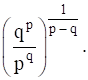4. If the pth and qth terms of a G.P. are q and p respectively, show that its (p + q)th term is

Solution: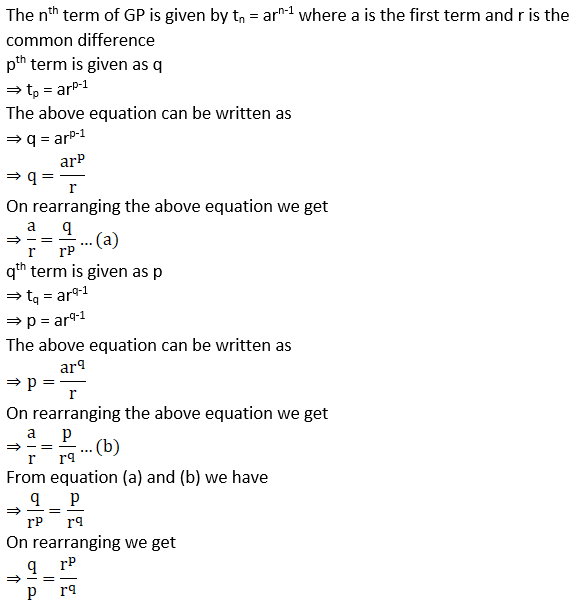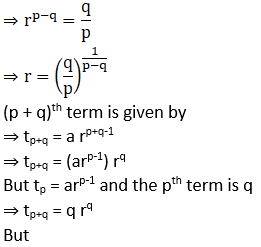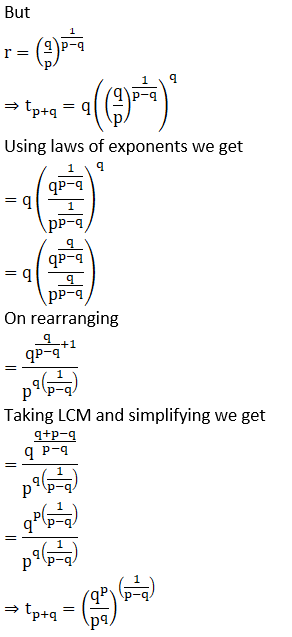Hence the proof.

5. A carpenter was hired to build 192 window frames. The first day he made five frames and each day, thereafter he made two more frames than he made the day before. How many days did it take him to finish the job?

Solution:

Given first day he made 5 frames then two frames more than the previous that is 7 then 9 and so on

Hence the sequence of making frames each day is 5, 7, 9…

The sequence is AP with first term as a = 5 and common difference d = 2

Total number of frames to be made is 192

Let is requires n days hence Sn = 192

The sum of first n terms of AP is given by Sn = n/2 (2a + (n – 1) d)

Where a is the first term and d is common difference

Sn = (n/2) (2(5) + (n – 1) 2)

192 = (n/2) (10 + 2n – 2)

⇒ 384 = 10n + 2n2 – 2n

On computing and simplifying we get

⇒ 2n2 + 8n – 384 = 0

⇒ n2 + 4n – 192 = 0

⇒ n2 + 16n – 12n – 192 = 0

⇒ n (n + 16) – 12 (n + 16) = 0

⇒ (n – 12) (n + 16) = 0

⇒ n = 12 and n = -16

But n represents number of days which cannot be negative hence n = 12

Hence number of days required to finish the job is 12 days.

6. We know the sum of the interior angles of a triangle is 180°. Show that the sums of the interior angles of polygons with 3, 4, 5, 6, … sides form an arithmetic progression. Find the sum of the interior angles for a 21-sided polygon.

Solution:

Given the sum of interior angles of a polygon having ‘n’ sides is given by (n – 2) × 180°

Sum of angles with three sides that is n = 3 is (3 – 2) × 180° = 180°

Sum of angles with four sides that is n = 4 is (4 – 2) × 180° = 360°

Sum of angles with five sides that is n = 5 is (5 – 2) × 180° = 540°

Sum of angles with six sides that is n = 6 is (6 – 2) × 180° = 720°

As seen as the number of sides increases by 1 the sum of interior angles increases by 180°

Hence the sequence of sum of angles as number of sides’ increases is 180°, 360°, 540°, 720° …

The sequence is AP with first term as a = 180° and common difference as d = 180°

We have to find sum of angles of polygon with 21 sides

Using (n – 2) × 180°

⇒ Sum of angles of polygon having 21 sides = (21 – 2) × 180°

⇒ Sum of angles of polygon having 21 sides = 19 × 180°

⇒ Sum of angles of polygon having 21 sides = 3420°

7. A side of an equilateral triangle is 20cm long. A second equilateral triangle is inscribed in it by joining the mid points of the sides of the first triangle. The process is continued as shown in the accompanying diagram. Find the perimeter of the sixth inscribed equilateral triangle.

Solution: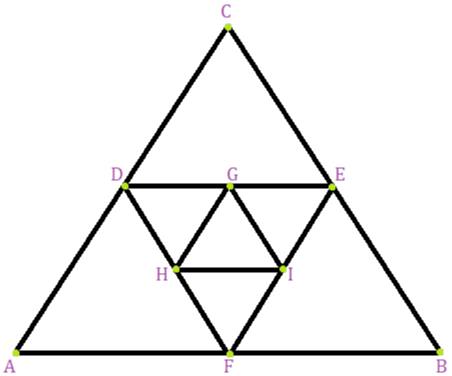Let ABC be the triangle with AB = BC = AC = 20 cm

Let D, E and F be midpoints of AC, CB and AB respectively which are joined to form an equilateral triangle DEF

Now we have to find the length of side of ΔDEF

Consider ΔCDE

CD = CE = 10 cm … D and E are midpoints of AC and CB

Hence ΔCDE is isosceles

⇒ ∠CDE = ∠CED … base angles of isosceles triangle

But ∠DCE = 60° …∠ABC is equilateral

Hence ∠CDE = ∠CED = 60°

Hence ΔCDE is equilateral

Hence DE = 10 cm

Similarly, we can show that GH = 5 cm

Hence the series of sides of equilateral triangle will be 20, 10, 5 …

The series is GP with first term a = 20 and common ratio r = ½

To find the perimeter of 6th triangle inscribed we first have to find the side of 6th triangle that is the 6th term in the series

nth term in GP is given by tn = arn-1

⇒ t6 = (20) (1/2)6-1

⇒ t6 = 20/ 25

= 20/ (4 × 23)

⇒ t6 = 5/8

Hence the side of 6th equilateral triangle is 5/8 cm and hence its perimeter would be thrice its side length because it’s an equilateral triangle

Perimeter of 6th equilateral triangle inscribed is 3 × 5/8 = 15/8 cm

8. In a potato race 20 potatoes are placed in a line at intervals of 4 metres with the first potato 24 metres from the starting point. A contestant is required to bring the potatoes back to the starting place one at a time. How far would he run in bringing back all the potatoes?

Solution:

Given at start he has to run 24m to get the first potato then 28 m as the next potato is 4m away from first and so on

Hence the sequence of his running will be 24, 28, 32 …

There are 20 terms in sequence as there are 20 potatoes

Hence only to get potatoes from starting point he has to run

24 + 28 + 32 + … up to 20 terms

This is only from starting point to potato but he has to get the potato back to starting point hence the total distance will be twice that is

Total distance ran = 2 × (24 + 28 + 32…) … 1

Let us find the sum using he formula to find sum of n terms of AP

That is Sn = n/2 (2a + (n – 1) d)

There are 20 terms hence n = 20

⇒ S20 = (20/2) (2 (24) + (20 – 1) 4)

On simplification we get

⇒ S20 = 10(48 + 19(4))

⇒ S20 = 10(48 + 76)

On computing we get

⇒ S20 = 10 × 124

⇒ S20 = 1240 m

Using equation 1

⇒ Total distance ran = 2 × 1240

⇒ Total distance ran = 2480 m

Hence total distance he has to run is 2480 m

9. In a cricket tournament 16 school teams participated. A sum of Rs 8000 is to be awarded among themselves as prize money. If the last placed team is awarded Rs 275 in prize money and the award increases by the same amount for successive finishing places, how much amount will the first place team receive?

Solution:

Let the amount received by first place team be a Rs and d be difference in amount

As the difference is same hence the second-place team will receive a – d and the third place a – 2d and so on

The last team receives 275 Rs

As there are 16 teams and all teams are given prizes hence the sequence will have 16 terms because there are 16 teams

Therefore a, a – d, a – 2d… 275

The sequence is in AP with first term as a and common difference is ‘-d’

As the total prize given is of 8000rs hence

a + a – d + a – 2d … + 275 = 8000

The sum of n terms of AP is given by Sn = n/2 (2a + (n – 1) d) where

A is first term and d is common difference

There are 16 terms n = 16 and the sum Sn = 8000

⇒ 8000 = (16/2) (2a + (16 – 1) (-d))

⇒ 8000 = 8(2a – 15d)

⇒ 1000 = 2a – 15d ……1

The last term of AP is 275 and nth term of AP is tn = a + (n – 1) d

The last term is tn = 275

⇒ 275 = a + (16 – 1) (-d)

⇒ 275 = a – 15d ……2

Subtract 2 from 1 that is 1 – 2

⇒ 1000 – 275 = 2a – 15d – a + 15d

⇒ 725 = a

Hence amount received by first place team is Rs.725

10. If a1, a2, a3, …, an are in A.P., where ai > 0 for all i, show that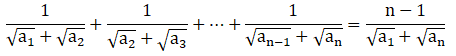Solution: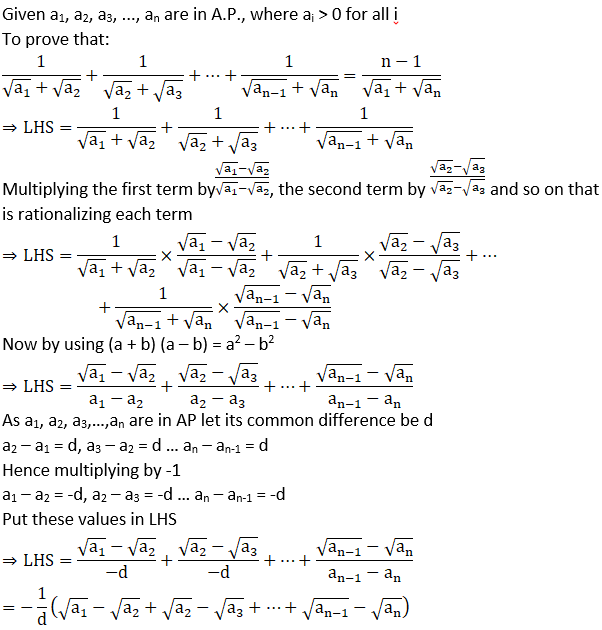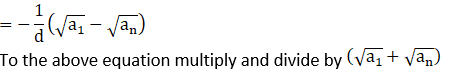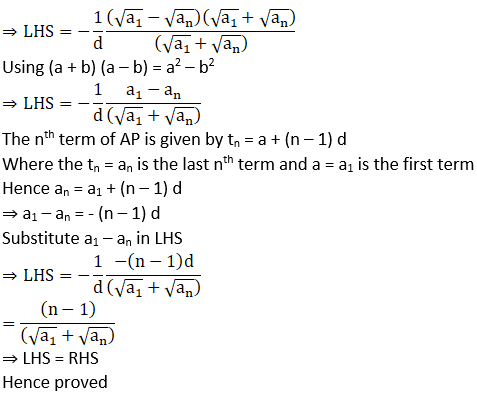11. Find the sum of the series (33 – 23) + (53 – 43) + (73 – 63) + … to

(i) n terms

(ii) 10 terms

Solution: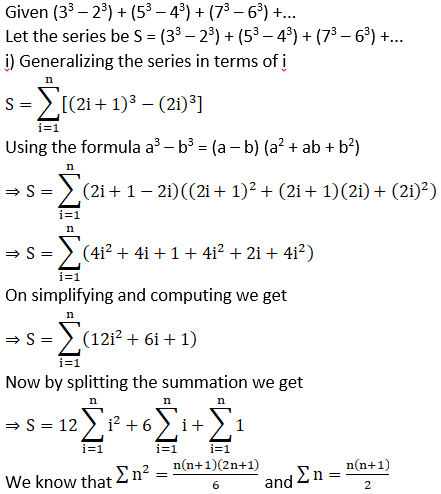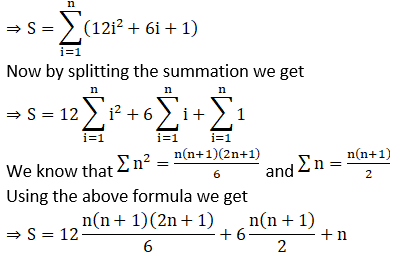Simplifying we get

⇒ S = 2n (n+1) (2n+1) + 3n (n+1) + n

⇒ S = 2n (2n2 + 2n + n + 1) + 3n2 + 3n + n

⇒ S = 4n3 + 6n2 + 2n + 3n2 + 4n

⇒ S = 4n3 + 9n2 + 6n

Hence sum up to n terms is 4n3 + 9n2 + 6n

ii) Sum of first 10 terms or up to 10 terms

To find sum up to 10 terms put n = 10 in S

⇒ S = 4 (10)3 + 9 (10)2 + 6 (10)

⇒ S = 4000 + 900 + 60

⇒ S = 4960

Hence sum of series up to 10 terms is 4960

12. Find the rth term of an A.P. sum of whose first n terms is 2n + 3n2.
[Hint: an = Sn – Sn–1]

Solution:

Sum of first n terms be Sn given as Sn = 2n + 3n2

We have to find the rth term that is ar

Using the given hint nth term is given as an = Sn – Sn-1

⇒ ar = Sr – Sr-1

Using Sn = 2n + 3n2

⇒ ar = 2r + 3r2 – (2(r – 1) + 3(r – 1)2)

⇒ ar = 2r + 3r2 – (2r – 2 + 3(r2 – 2r + 1))

⇒ ar = 2r + 3r2 – (2r – 2 + 3r2 – 6r + 3) ⇒ ar = 6r – 1

Hence the rth term is 6r – 1

13. If A is the arithmetic mean and G1, G2 be two geometric means between any two numbers, then prove that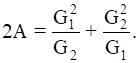Solution: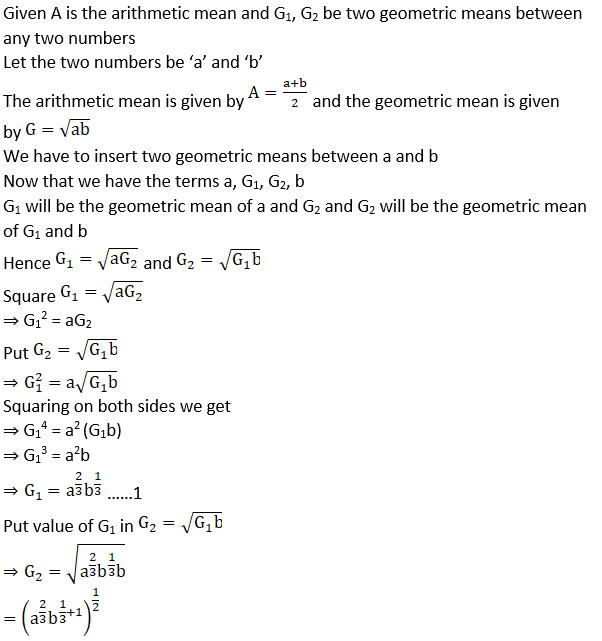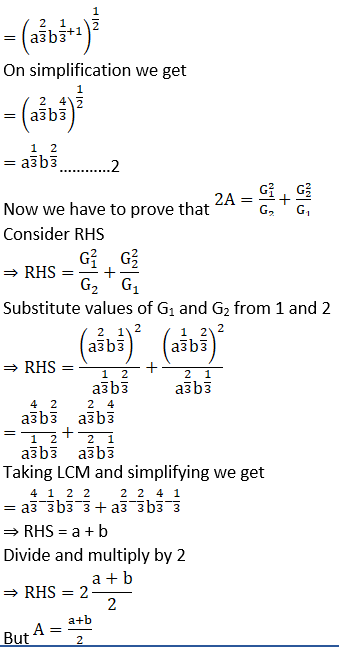Therefore

⇒ RHS = 2A

Hence RHS = LHS

Hence proved

14. If θ1, θ2, θ3, …, θn are in A.P., whose common difference is d, show that Sec θ1 sec θ2 + sec θ2 sec θ3 + … + sec θn–1 sec θn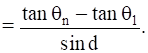Solution: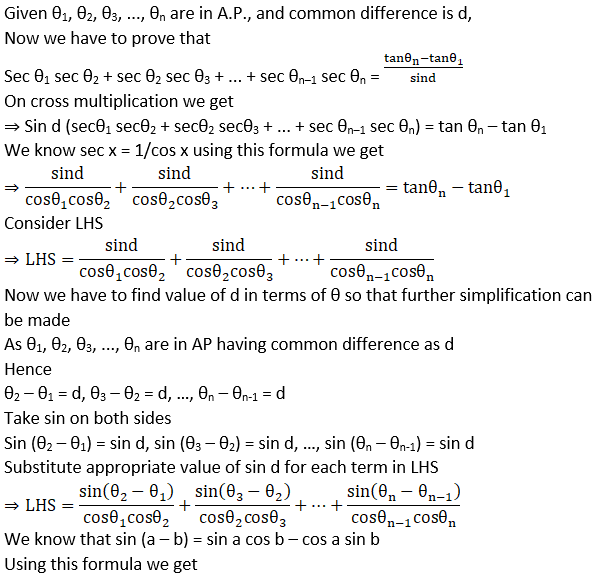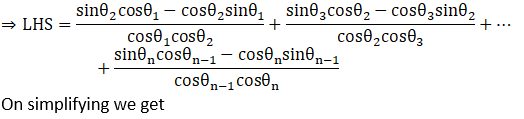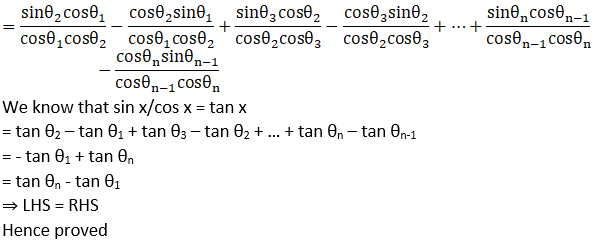15. If the sum of p terms of an A.P. is q and the sum of q terms is p, show that the sum of p + q terms is – (p + q). Also, find the sum of first p – q terms (p > q).

Solution: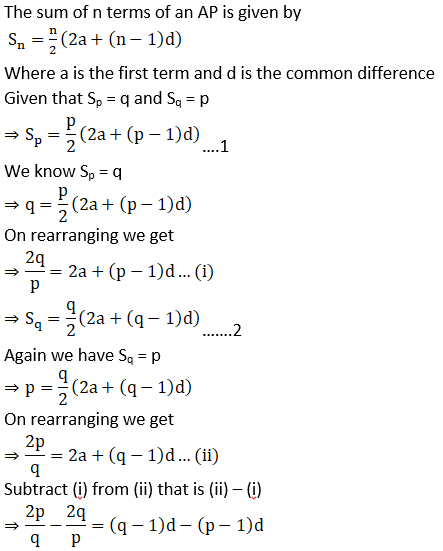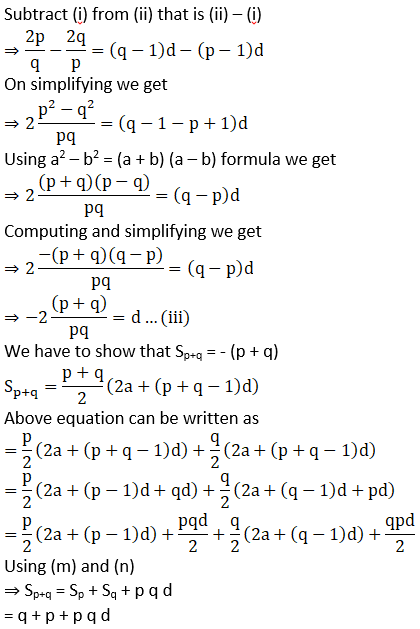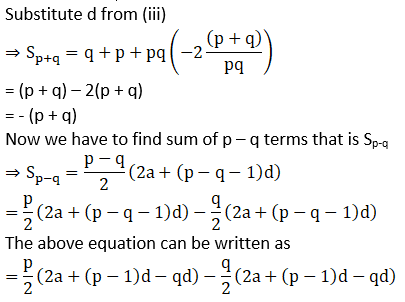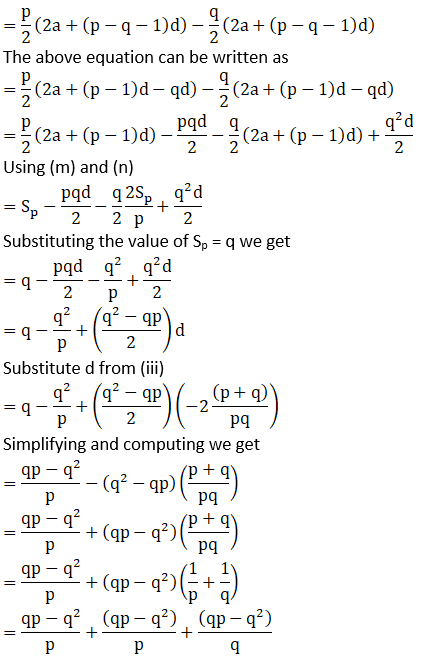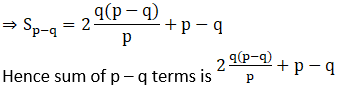16. If pth, qth, and rth terms of an A.P. and G.P. are both a, b and c respectively, show that

ab–c . bc – a. ca – b = 1

Solution: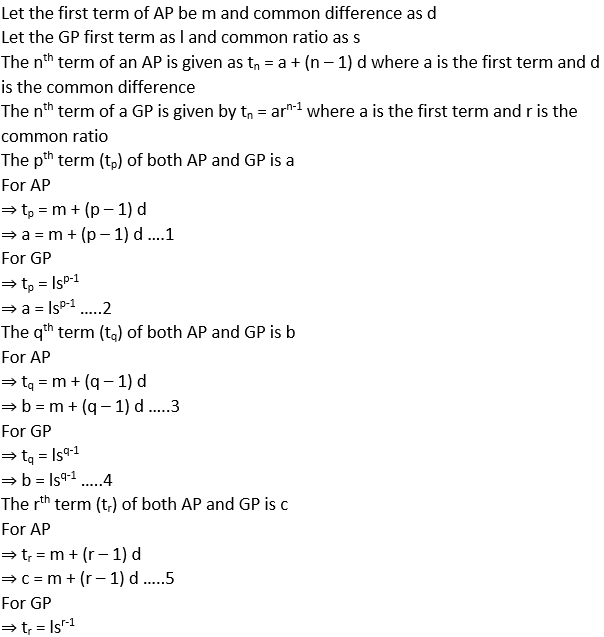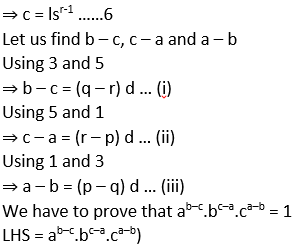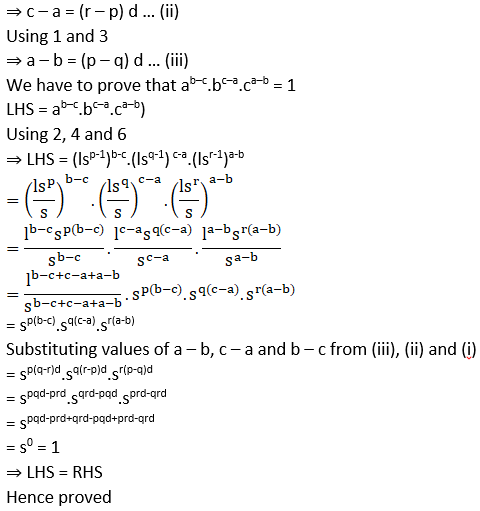Objective Type Questions

17. If the sum of n terms of an A.P. is given by Sn = 3n + 2n 2, then the common difference of the A.P. is

(A) 3 (B) 2 (C) 6 (D) 4

Solution:

(D) 4

Explanation:

To find: Common Difference of A.P that is d

Consider,

Sn = 3n + 2n2

Putting n = 1, we get

S1 = 3(1) + 2(1)2

= 3 + 2

S1 = 5

Putting n = 2, we get

S2 = 3(2) + 2(2)2

= 6 + 2(4)

= 6 + 8

S2 = 14

Now, we know that,

S1 = a1

⇒ a1 = 5

And a2 = S2 – S1

= 14 – 5

= 9

∴ Common Difference, d = a2 – a1

= 9 – 5

= 4

Hence, the correct option is (D)

18. The third term of G.P. is 4. The product of its first 5 terms is

(A) 43 (B) 44 (C) 45 (D) None of these

Solution:

(C) 45

Explanation:

Given the third term of G.P, T3 = 4

To find the product of first five terms

We know that,

Tn = a rn – 1

It is given that, T3 = 4

⇒ ar3 – 1 = 4

⇒ ar2 = 4 … (i)

Product of first 5 terms = a × ar × ar2 × ar3 × ar4

= a5r1+2+3+4

= a5r10

= (ar2)5

= (4)5 [from (i)]

Hence, the correct option is (C)